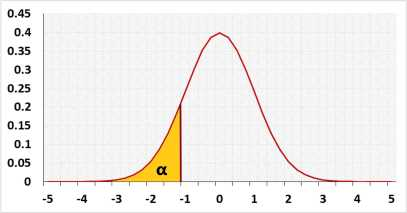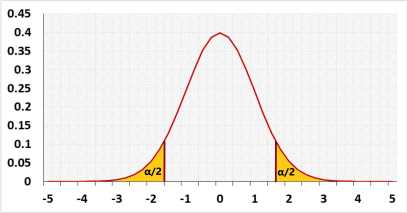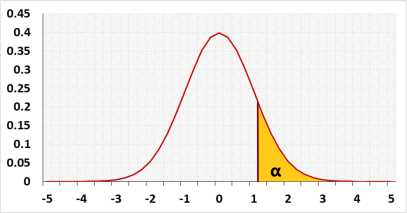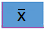# One Sample Z-Test Calculator

Known standard deviation
or or enter summarized data (x̅, n, σ, S) below

## Enter sample data

Header: You may change groups' name to the real names.
Data: When entering data, press Enter after each value.

The tool will not count empty cells or non-numeric cells.

## Enter sample data

You may copy data from Excel, Google sheets or any tool that separate data with Tab and Line Feed.
Copy the data, one block of 2 consecutive columns includes the header, and paste below.

Copy the data,

It is okay to leave empty cells, empty cells or non numeric cells won't be counted

1 - Not mandatory, uses to validate σ

The difference between the expected SD and the sample SD

When entering raw data, the tool will run the Shapiro-Wilk normality test and calculate outliers, as part of the test calculation.
validation message

## Information

Target: To check if the assumed μ0 is statistically correct, based on a sample average

Example1: A farmer calculated last year the average of the apples' weight in his apple orchard μ0 equals 17 kg, based on big sample
Current year he checked a small sample of apples and the sample average x equals 18 kg
Was the average of the apple's weight changed this year?

Hypotheses
H0: μ = μ0
H1: μ < > μ0
Test statisticNormal distribution## AssumptionsNormal distributionThe standard deviations of the population is knownPopulation expected mean is known

## Required Sample DataSample averageSample size

## R Code

The following R code should produce the same results:

Currently, there is no direct R function for the one-sample z test.

Examples
1. Two-tailed test
A farmer calculated last year the average of the apples' weight in his apple orchard μ0 equals 17kg, based on a big sample.
The current year the sample average x̅ equals 16kg.
Was the average of the apple's weight in the entire orchard changed this year? or is it just a random difference?

2. Left tail test.
In the same example as above, the farmer only cares if the entire average is lesser this year.